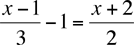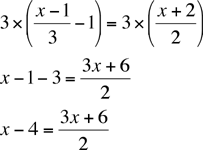Quandaries and Queries Who is asking: Student Level: Secondary Question:  (x-1)/3 - 1 = (x + 2)/2 Hi, You have two fractions, one with a denominator of 3 and the other with a denominator of 2. The first thing I would do is to multiply both sides by 3 as this will eliminate one of the fractions.Multiplying both sides by 3 and simplifying givesNow you have only one fraction, with a denominator of 2. Multiply both sides by 2, simplify and solve for x. Make sure you check your answer, Penny Go to Math Central